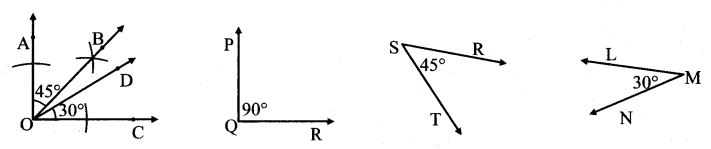# Maharashtra Board Class 7 Maths Solutions Chapter 1 Geometrical Constructions Practice Set 7

## Maharashtra State Board Class 7 Maths Solutions Chapter 1 Geometrical Constructions Practice Set 7

Question 1.
Some angles are given below. Using the symbol of congruence write the names of the pairs of congruent angles in these figures.Solution:
i. ∠AOC ≅ ∠PQR
ii. ∠DOC ≅ ∠LMN
iii. ∠AOB ≅ ∠BOC ≅ ∠RST

Maharashtra Board Class 7 Maths Chapter 1 Geometrical Constructions Practice Set 7 Intext Questions and Activities

Question 1.
Observe the given angles and write the names of those having equal measures.
(Textbook pg. no. 8 and 9)Solution:
i. ∠ABC and ∠SPM
ii. ∠NIT and ∠SRI
iii. ∠PTQ and ∠RTS

Question 2.
Observe the image shown in the adjacent figure and answer the following questions. (Textbook pg. no. 9)

1. What time does this clock show?
2. What is the measure of the angle between its two hands?
3. At which other times is the angle between the hands congruent with this angle?Solution:

1. 3 o’ clock.
2. 90°.
3. 9 o’ clock.

Question 3.
Get bangles of different sizes but equal thickness and find the congruent ones among them. (Textbook pg. no. 10)
Solution:
[Students should attempt the above activities on their own.]

Question 4.
Find congruent circles in your surroundings. (Textbook pg. no. 10)
Solution:
[Students should attempt the above activities on their own.]

Question 5.
Take some round bowls and plates. Place their edges one upon the other to find pairs of congruent edges. (Textbook pg. no. 10)
Solution:
[Students should attempt the above activities on their own.]